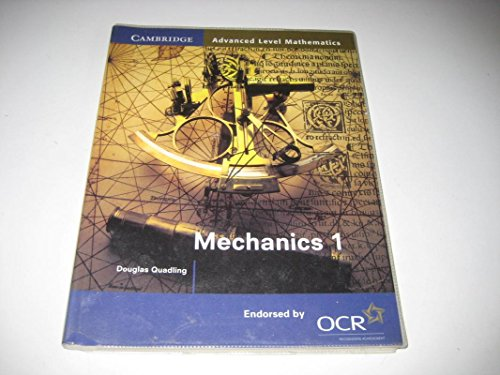Free Shipping on all orders in Australia
Over 7 million books in stock
Proud to be B-Corp# Pure Mathematics 1 and 2 by Hugh Neill

Condition - Very Good
\$25,99
8 in stock

## Summary

This book is part of the Cambridge Advanced Level Mathematics series, written specifically for the OCR modular mathematics syllabus. Pure Mathematics 1 & 2 contains the material for modules P1 and P2 in separate halves of the book. It has chapters on algebra, functions, graphs, trigonometry and exponentials, and it introduces calculus.

## Pure Mathematics 1 and 2 Summary

### Pure Mathematics 1 and 2 by Hugh Neill

This book is part of the Cambridge Advanced Level Mathematics series, written specifically for the OCR modular mathematics syllabus. Each book or half-book corresponds exactly to one module. Pure Mathematics 1 & 2 contains the material for modules P1 and P2 in separate halves of the book. It has chapters on algebra, functions, graphs, trigonometry and exponentials, and it introduces calculus. Each chapter starts with a short list of learning objectives, and mathematical ideas are explained carefully and clearly, with many worked examples. There are plenty of exercises throughout, along with revision exercises and mock exam papers - all written by experienced examiners.

Introduction; PURE MATHEMATICS 1: 1. Coordinates, points and lines; 2. Surds; 3. Some important graphs; 4. Quadratics ; 5. Differentiation ; 6. Inequalities; Revision exercises 1; 7. Index notation; 8. Functions and graphs; 9. Applications of differentiation; 10. Integration; 11. Trigonometry; 12. Second derivatives; Revision exercises 2; Mock examination papers; PURE MATHEMATICS 2: 1. Polynomials; 2. Combining and inverting functions; 3. Sequences; 4. The binomial theorem; 5. The trapezium rule; 6. Extending differentiation and integration; 7. Volumes of revolution; Revision exercises 3; 8. The logic of mathematics; 9. The modulus function; 10. Geometric sequences and exponential growth; 11. Exponential and logarithmic functions; 12. Differentiating exponentials and logarithms; 13. The chain rule; 14. Solving equations numerically; 15. Radians; Revision exercises 4; Mock examination papers; P1 Answers; P2 Answers; Index

GOR001239943
9780521783699
0521783690
Pure Mathematics 1 and 2 by Hugh Neill
Used - Very Good
Paperback
Cambridge University Press
2000-05-08
412
N/A
Book picture is for illustrative purposes only, actual binding, cover or edition may vary.
This is a used book - there is no escaping the fact it has been read by someone else and it will show signs of wear and previous use. Overall we expect it to be in very good condition, but if you are not entirely satisfied please get in touch with us